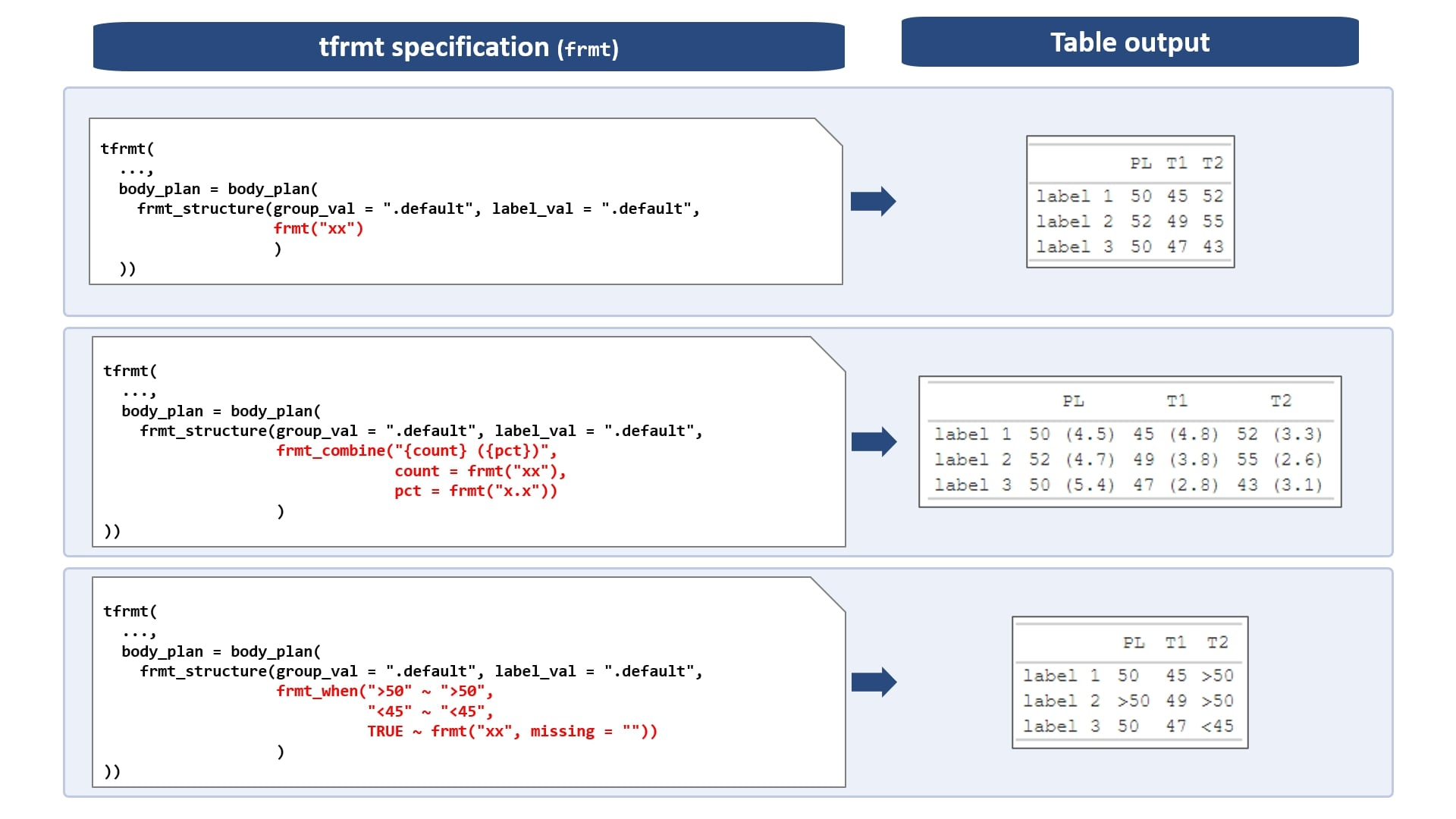The body plan covers the formatting of numeric cell values. Within the body plan, format structures (frmt_structure) may be layered to specify formatting for different parameters. Formatting can be applied to specific group and/or label values, which we will cover in a bit. First, we will apply formats to all relevant values in the table, regardless of group or label value.

## Formatting functions

The following formatting functions may be passed to frmt_structure:

### Format (frmt)

The frmt object is used to specify how a value should appear in a table: its number of digits, any white space padding, or extra characters, such as percentages or parentheses. In pharma, it is a common convention to use x’s to represent data values in mock tables. As such, the frmt complies with this familiar convention. For example:

• frmt(expression = "xxx.x%") displays 3.829765 as ” 3.8%” (note the leading whitespace!)
• frmt(expression = "xxx.x%", missing = "Missing") displays NA as “Missing”
• frmt(expression = "xxx.x", scientific = " x10^x") displays 1234.5678 as ” 1.2 x10^ 3”

### Format when (frmt_when)

frmt_when is a way to format cells conditional on their values. frmt_when is similar to dplyr::case_when or if/else statements. For each condition, the left hand side is an expression as a string and the right hand side is the formatting to be applied. For example, the following code allows users to conditionally format the upper and lower bounds for p-values:

frmt_when(
">0.99" ~ ">0.99",
"<0.001" ~ "<0.001",
TRUE ~ frmt("x.xxx")
)

This will cause the values 0.0347, 1.00, and 0.000001 to appear as “0.035”, “>0.99”, and “<0.001”.

### Format combine (frmt_combine)

frmt_combine is used to combine multiple values into a single cell. Within a frmt_combine, the values are referenced according to the values of the param variable. Glue package syntax is leveraged to help the user easily define how they want the combined values to appear. Further, the individual data values may be formatted using frmt or frmt_when. Some examples:

• frmt_combine("({lower}, {upper})", lower = frmt("xx.x"), upper = frmt("xx.x")) will combine lower and upper bounds of a confidence interval into: “(xx.x, xx.x)”.

• frmt_combine("{n} ({pct})", n = frmt("xxx"), pct = frmt("xx.x", missing = "")) will print “n (%)” if the percentage is non-missing, but just “n” otherwise.

• frmt_combine("{n} ({pct})", n = frmt("xxx"), pct = frmt("xx.x", missing = ""), missing = "") will print “n (%)” if the percentage is non-missing, “n” if the percentage is missing but n is non-missing, and “” if both n and pct are missing.

## Location options

There are a variety of ways for the user to specify formatting locations: group variable values and/or label values, or by param value. Let’s take a look at the various methods below, ranging from least specific to most specific.

An important detail is that passing a value of “.default” to group or label means that the format will be applied to ALL values within the group(s) or label variables.

### Table-wide

A user can specify formatting for the entire table as such:

frmt(group = ".default", label = ".default", frmt("xx.x"))

### Param-specific

For formatting specific values of the param variable, frmt objects must be named according to the param. The following code will format all values where the param value equals “pval”, regardless of group, label, or column value.

frmt(group = ".default", label = ".default", pval = frmt("x.xxx"))

Again, param names are required for using frmt_combine.

### Label-specific

For formatting specific values of the label variable, these values are passed to the label argument. The following code will format all values where the label variable equals “mylab1” or “mylab2”, regardless of group, param, or column value.

frmt(group = ".default", label = c("mylab1","mylab2"), frmt("x.xxx"))

### Group-specific

For formatting specific values of the group variable, these values are passed to the group argument. Note that the user may have multiple group variables. In the case of multiple group variables, the specifications are to be passed as a named list (named according to the group variables). The following code will format all values where group1=“A” and group2=“B”, regardless of label, param, or column value:

frmt(group = list(group1 = "A", group2 = "B"), label = ".default", frmt("x.xxx"))

### Column-specific

In the case where formatting needs to differ between columns within a given group/label/param level, the user will need to incorporate column-level information into the param value. For example, if we have two columns, col1 and col2, but we want the mean for col1 to be rounded differently than col2, creating our param column as such will allow us to differentiate the mean values in the formatting: param = c("col1_mean", "col2_mean").

### Multiple specifications

A user can be as specific as they’d like, by providing any of these in combination. For example, the following code will format values where group1=“A”, group2=“B”, the label variable equals “mylab” and the param variable equals “mean”:

frmt(group = list(group1 = "A", group2 = "B"), label = "mylab", mean = frmt("x.xxx"))

NOTE: the tfrmt_sigdig() function provides the ability to create a tfrmt with auto-precision based on the significance of the group/label value.

## Layering format structures

The user may provide as many frmt_structure objects as needed. It is important to note that if there are overlapping frmt_structure objects, the last one specified will supersede the previous. In our CDISC demographics table example, the following frmt_structures are needed to cover all values:

body_plan(
frmt_structure(group_val = ".default", label_val = ".default",
frmt_combine("{n} {pct}",
n = frmt("XXX"),
pct = frmt_when("==100" ~ "",
"==0" ~ "",
TRUE ~ frmt("(XX.X %)"))
)
),
frmt_structure(group_val = ".default", label_val = "n", frmt("xxx")),
frmt_structure(group_val = ".default", label_val = c("Mean", "Median", "Min","Max"), frmt("xxx.x")),
frmt_structure(group_val = ".default", label_val = "SD", frmt("xxx.xx")),
frmt_structure(group_val = ".default", label_val = ".default", p = frmt_when(">0.99" ~ ">0.99",
"<0.001" ~ "<0.001",
TRUE ~ frmt("x.xxx", missing = "")))
)

### Help images